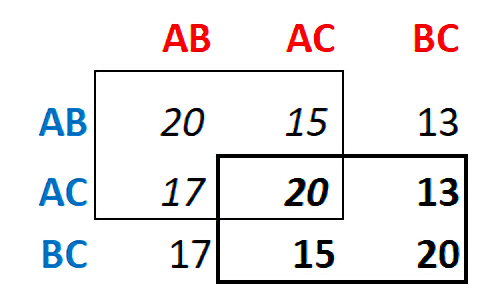BIOL4250 - Midterm Questions for 2021, as of Oct 12, for exam on 2021 October 28

Prepare answers to ALL of the HOMEWORK questions below. For the exam, I will choose FOUR: you must answer any THREE in the exam Period. Show your work.

[I suggest that you not use calculators]

1.       Prior to the advent of molecular data in the 1960s, it was assumed that the large organismal differences between humans relative to chimps and gorillas were due to a large amount of genetic change along the human lineage. Test this hypothesis by counting the number of SNP changes between the three pairwise combinations of Homo sapiensPan troglodytes, and Gorilla gorilla. Report these numbers: do the data support or reject the hypothesis? Explain.

2.       William J Spillman was an American agronomist who in 1901 independently observed experimentally what would later be called Mendelian ratios. Answer the HOMEWORK question here on his crosses.

3.       We showed in class that with n = 50, a ratio of 29:21 is insufficient to demonstrate a significant deviation from an expected 25:25 ratio. With n=50, what is the minimum deviation from expectation that could be detected as statistically significant? From the formula for Chi-Square, and given a critical value of X2 = 3.84, show algebraically what that minimum deviation is.

4.       Calculate Hexp for a locus with 10 alleles at equal frequency: show your work. How many genotypes are there at such a locus? Answer the same questions, for 100 alleles at equal frequency.

5.       Repeat the derivation of the Hardy-Weinberg Theorem in terms ofp = (p' - p).

6.       Demonstrate that the curves for positive directional selection can be obtained from appropriate values entered in the GSM model in Excel.

7.       For each of the four graphs, identify which mode of selection is acting on allele B, where q = f(B). Explain the features of q over time that allow you to recognize the mode of selection.

8.       Observe that the fitness curves for dominant and recessive alleles have the same shape, that is, if the green curve is flipped across the red curve, and flipped again along its length? Explain why.

9.       For a semi-dominant phenotype with Additive or Genic selection, for an initial f(A) < 0.01 and s < 0.5, use the GSM worksheet in Excel to run the (1) Additive and (2) Genic selection schemes in the table provided. At what values do the curves deviate and (or) converge on each other? Why?

10.   Professor Blue's midterm will have three questions, hardest (A), hard (B), and easy (C). She will ask any two of them. Student Red decides they can study for any two, but not all three. The student will get 10 points for a prepared answer, but only 3, 5, & 7 for unprepared answers to A, B, & C respectively. For the two 2x2 games in italics [upper left] or bold [lower right] below, calculate the optimal mixed strategies for Blue and Red, where matrix values are payoffs (test scores) to Red. Calculate the Value of the game to Red.
BONUS
: the matrix implicitly includes six other 2x2 games: what are they? Based on basic principles of game theory, why do none require mixed strategy solutions?All text material © 2021 by Steven M. Carr